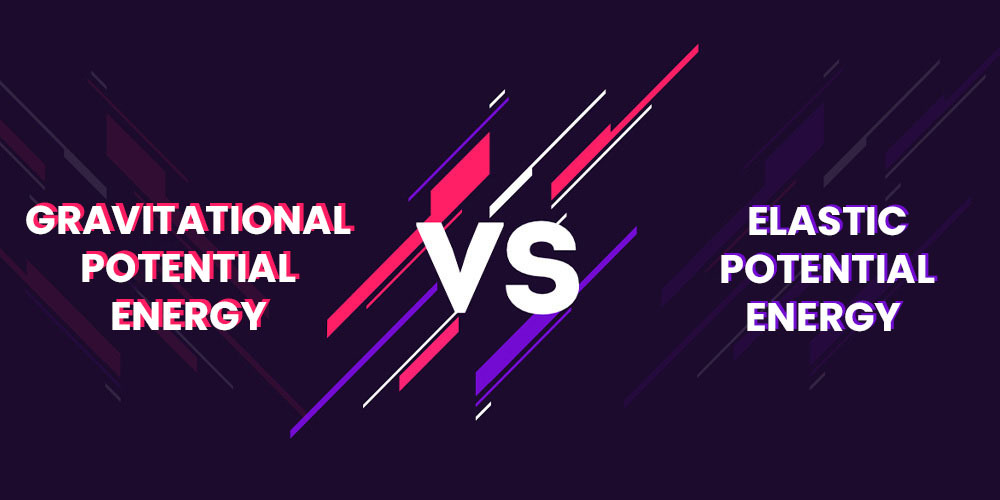GeeksforGeeks App
Open AppBrowser
Continue

# Difference between Gravitational Potential Energy and Elastic Potential Energy

Potential energy is defined as the energy stored in a body due to its physical properties like the mass of the object or position of the object. It is the force that a body could potentially develop when it is put into motion. There are different forms of potential energy, such as elastic potential energy, gravitational potential energy, electric (electromagnetic) potential energy, and nuclear potential energy. The potential energy of an object is directly proportional to the mass, height, or vertical position of the object.### What is Gravitational Potential Energy?

The gravitational potential energy of a particle at a point is defined as the work done by an external agent in moving the object from infinity to that point against the gravitational field. It is equal to the product of potential at the point due to the body and mass of the body.

For example, An object placed on a flat surface exhibits gravitational potential energy due to its position above the surface.

Formula of Gravitational Potential Energy:

Consider an object of mass m is lifted in a uniform gravitational field, through a height of h.

Therefore, the weight of the object, in this case, is given as,

w = mg

where m is the mass of the object and g is the acceleration due to gravity.

Now, if the body is lifted up with a uniform speed such that this work done required to lift the object up is provided to the object as gravitational potential energy (Eg). Also, as the object is lifted with uniform speed so both the lifting forces and normal forces are balanced by each other. This implies:

F = mg

Since the object is lifted to the height h therefore the work done is given as:

W = mgh

Also, the body is raised up at constant speed so the work done required to lift the body up is equal to the gain in gravitational potential energy of the body due to its rise.

Hence, the gravitational potential energy is,

Eg = mgh

Therefore,

• The SI unit of gravitational potential energy is J/kg (Joule-per-Kilogram).
• The dimensional formula of gravitational potential energy is [M0L2T-2].

### What is Elastic Potential Energy?

When an object is physically deformed, it gains elastic potential energy. The molecules that make up a material are pushed to move away from their equilibrium positions when an object is stretched. If the material is elastic, the molecules will attempt to return to their original positions. This allows the object to work effectively.

As a result, we say that an elastic material has elastic potential energy when it is deformed. When a spring or rubber band is stretched, for example, it gains elastic potential energy.

Formula of Elastic Potential Energy:

Since the force required to stretch a spring with the spring constant k to a displacement x is given by,

F = kx

Then the elastic potential energy in the spring system is,

U = ½ kx2

Therefore,

• The SI unit of Elastic Potential energy is Joule (J).

### Sample Problems

Problem 1: What is the gravitational potential energy of an object with a mass of 6.25 kgs. The distance between the objects is 340 m

Solution:

Given that,

The mass of object m = 6.25 kg,

Height, h = 340 m.

Since,

Eg = mgh

= 6.25 × 9.8 × 340

= 18.375 J

Problem 2: Two objects with masses 6 × 1020 kg and 3 × 109 kg are kept at a distance of 1200 km away from each other. Find the gravitational potential energy?

Solution:

Given that,

The mass of object 1 M = 6 × 1020 kg
The mass of object 2 m = 3 × 109 kg
The distance between them r = 1200 km

Since,

F = – GMm/r

= – 6.67 × 10-11 × 6 × 1020 × 3 × 109/1200000

= 10  × 1013

= 1014 J

Problem 3: Let a spring be elongated to 20 cm by an applied force from its equilibrium position with spring constant k = 0.1 N/m. Find the potential energy stored in spring?

Solution:

Given that,

Spring elongated x = 20 cm = 0.2 m

Spring constant k = 0.1 N/m

Potential energy stored in the spring is

U = 1/2 kx2

= 1/2 × 0.1 × (0.2)2

= 0.1 × 0.1 × 0.2

= 0.002 J

Problem 4: James stretches a spring with a spring constant of 150 N/m. He stretches the spring to 50 cm. What will be the elastic potential energy in the spring?

Solution:

Given that,

Spring elongated x = 50 cm = 0.5 m

Spring constant k = 150 N/m

Elastic Potential energy of the spring is

U = 1/2 kx2

= 1/2 × 150 × 0.5 × 0.5

= 18.75 J

Question 5: A wire is elongated from its original length of 4m to 6m with a spring constant of 200 N/m. Find the elastic potential energy stored in the spring?

Solution:

Given that,

Spring elongated x = 6 m – 4 m = 2 m

Spring constant k = 200 N/m

Potential energy stored in the spring is,

U = 1/2 kx2

= 1/2 × 200 × 2 × 2

= 400 J

My Personal Notes arrow_drop_up
Related Tutorials